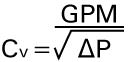# What is Cv and how do I use it to size valves?

Flow coefficient, commonly designated as Cv, is a measure of the resistance to flow. This factor must be measured using standardized flow tests specifically for this purpose, even though estimations can sometimes also be made by calculations alone. Once the factor is known, it is used in standardized equations to calculate flow or related parameters.where…
GPM = water flow in gallons/minute
P = pressure drop in psi